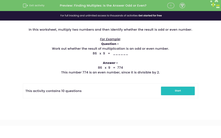# Finding Multiples: Is the Answer Odd or Even?

In this worksheet, students multiply two numbers together then state whether the answer is odd or even.Key stage:  KS 2

Curriculum topic:   Number: Multiplication and Division

Curriculum subtopic:   Multiply/Divide Formally

Popular topics:   Multiplication worksheets

Difficulty level:#### Worksheet Overview

In this worksheet, multiply two numbers and then identify whether the result is odd or even number.

For Example:

Question -
Work out whether the result of multiplication is an odd or even number.

86   x  9   =   ______

86   x  9   =  774

This number 774 is an even number, since it is divisible by 2.

### What is EdPlace?

We're your National Curriculum aligned online education content provider helping each child succeed in English, maths and science from year 1 to GCSE. With an EdPlace account you’ll be able to track and measure progress, helping each child achieve their best. We build confidence and attainment by personalising each child’s learning at a level that suits them.

Get started